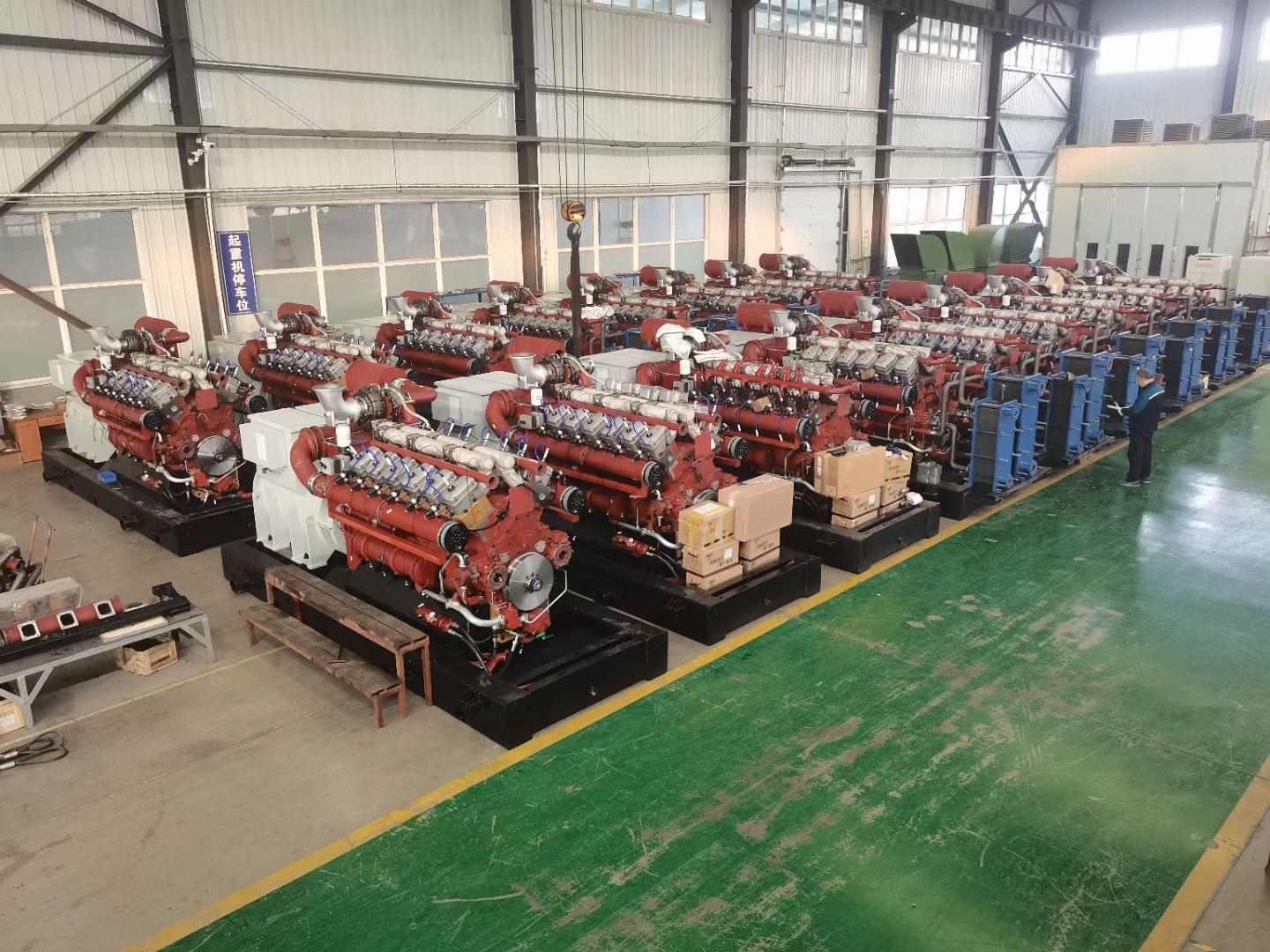全国服务热线:

0531-62325028济南济柴环能燃气发电设备有限公司

# 沼气发电机组总装机总量是多少？

When the raw material is stable and the production process is stable, the methane content and gas production of biogas will tend to be stable, and the fluctuation will not be too large. At this time, the total installed capacity of biogas generator unit can be determined.

The installed capacity of the power station shall be determined according to the biogas output and its low calorific value according to the formula:
P=k(V*H/G)
P=k(V*H/G)
P电站总装机容量，单位为kW
P Total installed capacity of the power station, in kW
k装机余量与发电机效率的综合比例系数，根据电站需求k=1.08~1.20
The comprehensive proportion coefficient of k installed margin and generator efficiency, according to the power station demand, k=1.08~1.20V每小时大沼气产量换算为标准状况下的体积，单位为m3/h
V Hourly biogas output is converted into volume under standard conditions, unit: m3/h
H沼气的低热值，单位为kJ/Nm3
H Low calorific value of biogas, in kJ/Nm3
G发电机组热耗率，单位为kJ/(kWh)
G Heat rate of generator unit, in kJ/(kWh)

Many of the above indicators are not easy to use. We need to change and move forward.
V，沼气产量，比较常见的对应参数是每天产量，即m3/d
5. The most common corresponding parameter of biogas output is the daily output, i.e. m3/d
H，沼气低热值，比较常见的对应参数是甲烷浓度
H. Methane concentration is a relatively common parameter corresponding to the low calorific value of biogas
G，发电机组热耗，比较常见的对应参数是发电机组发电效率
G. The heat consumption of the generator set, the relatively common corresponding parameter is the generation efficiency of the generator set

So the formula becomes as follows:
P=k((V/24)*(H*35800)/(35800/10/G)
P=k((V/24)*(H*35800)/(35800/10/G)

To simplify:
P=k(V*H*G*10)/24
P=k(V*H*G*10)/24

Note that Lao Liu is lazy here. The code in the other formula has not changed, but the meaning is completely different. You need to select the corresponding formula according to the actual situation.

Let's actually apply this formula. The biogas output is 10000 cubic meters per day; The general concentration of biogas is about 55% - 60%, here we take an integer of 60%; The efficiency of most imported biogas generating units is more than 40%, and the efficiency is not much worse, so we take 40%; The margin factor is ignored first

### 相关产品 / Related products

###### 济南济柴环能燃气发电设备有限公司
• 国内业务:高经理18653152096
王经理13012995645
• 国外业务:李经理18668968402
张经理18678860619
• 座 机：0531-62325028 / 69951266
• 传 真：0531-69958299
• 邮 箱：hengnenghr@163.com
• 地 址：济南市高新区舜华路2000号舜泰广场9号楼南楼201打开微信